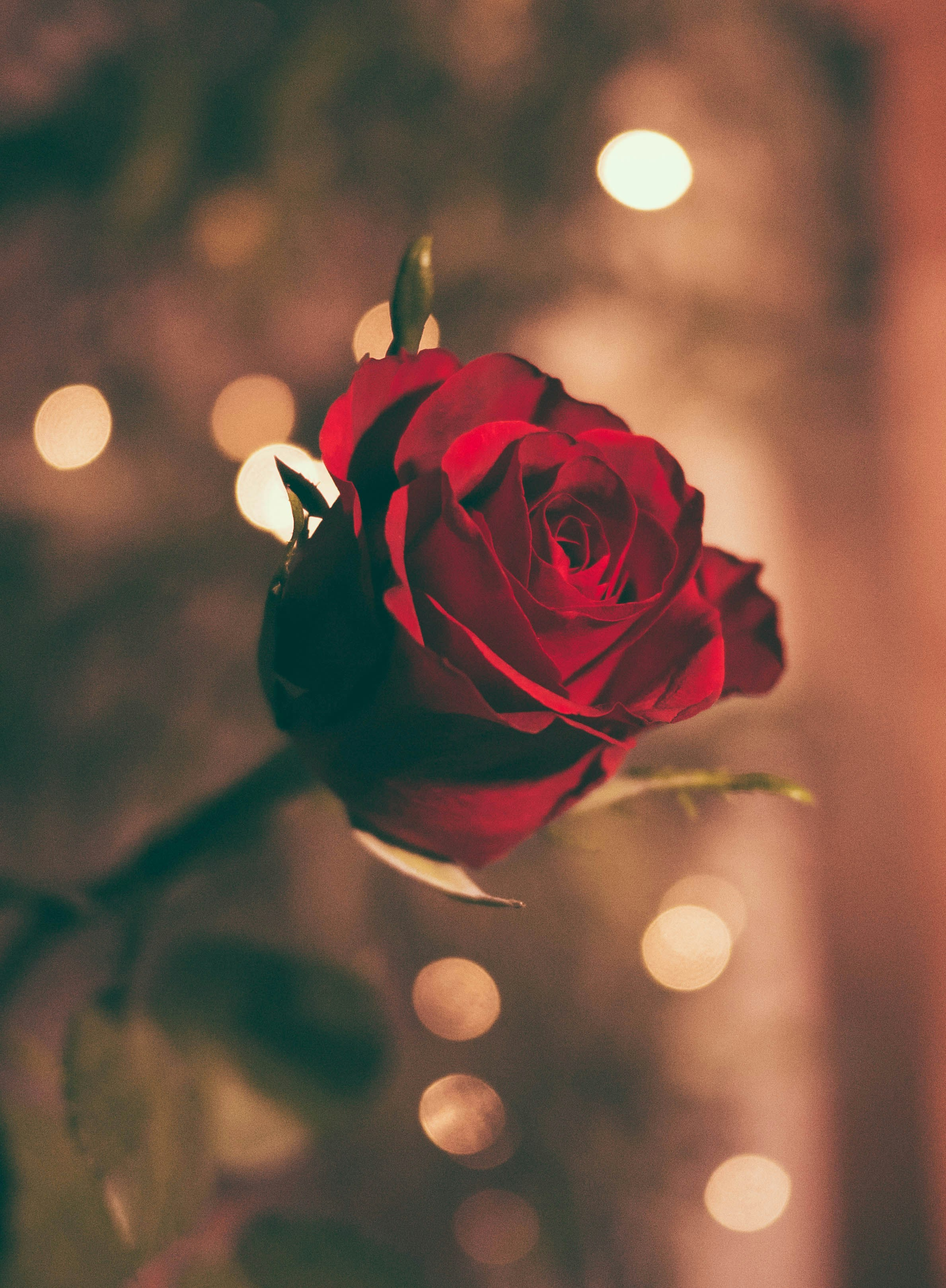# Rosen sind rot

Pussy

Roses are red, violets are blue, vodka costs less than dinner for two.Roses are red, violets are blue, vodka costs less than dinner for two.Roses are red, violets are blue, vodka costs less than dinner for two.Roses are red, violets are blue, vodka costs less than dinner for two.Roses are red, violets are blue, vodka costs less than dinner for two.Roses are red, violets are blue, vodka costs less than dinner for two.Roses are red, violets are blue, vodka costs less than dinner for two.Roses are red, violets are blue, vodka costs less than dinner for two.Roses are red, violets are blue, vodka costs less than dinner for two.Roses are red, violets are blue, vodka costs less than dinner for two.Roses are red, violets are blue, vodka costs less than dinner for two.Roses are red, violets are blue, vodka costs less than dinner for two.Roses are red, violets are blue, vodka costs less than dinner for two.Roses are red, violets are blue, vodka costs less than dinner for two.Roses are red, violets are blue, vodka costs less than dinner for two.Roses are red, violets are blue, vodka costs less than dinner for two.Roses are red, violets are blue, vodka costs less than dinner for two.Roses are red, violets are blue, vodka costs less than dinner for two.Roses are red, violets are blue, vodka costs less than dinner for two.Roses are red, violets are blue, vodka costs less than dinner for two.Roses are red, violets are blue, vodka costs less than dinner for two.Roses are red, violets are blue, vodka costs less than dinner for two.Roses are red, violets are blue, vodka costs less than dinner for two.Roses are red, violets are blue, vodka costs less than dinner for two.Roses are red, violets are blue, vodka costs less than dinner for two.Roses are red, violets are blue, vodka costs less than dinner for two.Roses are red, violets are blue, vodka costs less than dinner for two.Roses are red, violets are blue, vodka costs less than dinner for two.Roses are red, violets are blue, vodka costs less than dinner for two.Roses are red, violets are blue, vodka costs less than dinner for two.Roses are red, violets are blue, vodka costs less than dinner for two.Roses are red, violets are blue, vodka costs less than dinner for two.Roses are red, violets are blue, vodka costs less than dinner for two.Roses are red, violets are blue, vodka costs less than dinner for two.Roses are red, violets are blue, vodka costs less than dinner for two.Roses are red, violets are blue, vodka costs less than dinner for two.Roses are red, violets are blue, vodka costs less than dinner for two.Roses are red, violets are blue, vodka costs less than dinner for two.Roses are red, violets are blue, vodka costs less than dinner for two.Roses are red, violets are blue, vodka costs less than dinner for two.Roses are red, violets are blue, vodka costs less than dinner for two.Roses are red, violets are blue, vodka costs less than dinner for two.Roses are red, violets are blue, vodka costs less than dinne

Anzeige
Anzeige

Liebe

Willkommen

Anzeige
Anzeige

# Rap

Anonym

Deutsch rap ist früher denn je

Anzeige